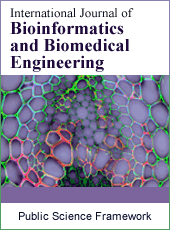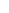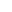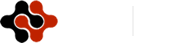International Journal of Bioinformatics and Biomedical Engineering
Articles Information
International Journal of Bioinformatics and Biomedical Engineering, Vol.1, No.2, Sep. 2015, Pub. Date: Jul. 15, 2015
Robust Numerical Solution of the Time-Dependent Problems with Blow-Up
 Kolade M. Owolabi, Department of Mathematical Sciences, Federal University of Technology, Ondo-State, Nigeria; Department of Mathematics and Applied Mathematics, University of the Western Cape, Bellville, Republic of South Africa.
Numerical solutions of nonlinear time-dependent partial differential equations with blow-up are considered in this paper. Such systems of PDEs are categorized into linear and nonlinear parts to allow the use of two classic mathematical ideas in space and time. The main focus in this paper is to discretized in space with higher order finite difference approximation and integrate the resulting nonlinear ordinary differential equations with an adaptive fourth-order exponential time differencing Runge-Kutta (ETDRK4) scheme. Stability analysis of the scheme is also examined. This paper is primarily concerned with the use of the ETDRK4 method to simulate some of the blow-up phenomena in nonlinear parabolic equations that are largely encountered in a number of physical situations, like chemical reaction-diffusion, electrical heating, fluid flow and population growth. It is expected that the time at which blow-up occurs will reflect in the numerical results.
Blow-Up Problems, Exponential Time Differencing Methods, Nonlinear, Time-Dependent Pdes, Stability
 J. Bebernes, D. Eberly, Mathematical Problems from Combustion Theory, Applied Mathematical Science, Springer, New York, 1989.
 G. Beylkin, J.M. Keiser and L. Vozovoi, A new class of time discretization schemes for the solution of nonlinear PDEs, Journal of Computational Physics 147 (1998) 362-387.
 H. Bernard, A. Islas and C.M. Schober, Conservation of phase properties using exponential integrators on the cubic Scr¨odinger equation, Journal of Computational Physics 225 (2007) 284-299.
 P. L. Bhatnagar, Nonlinear waves in one-dimensional dispersive systems, Oxford Mathematical Monographs, 1979.
 J. P. Boyd, Chebyshev and Fourier Spectral Methods, Dover, New York, 2001.
 C. J. Budd, G. J. Collins and V.A. Galaktionov, An asymptotic and numerical description of semi-linear blow-up in quasilinear parabolic equations, Journal of Computational and Applied Mathematics 97 (1998) 51-80.
 S. M. Cox and P.C. Matthews, Exponential time differencing for stiff systems, Journal of Computational Physics 176 (2002) 430-455.
 F. de la Hoz and F. Vadilo, An exponential time differencing method for the nonlinear schrodinger equation, Computer Physics Communications 179 (2008) 449-456.
 G. Filippas and R. V.Kohn, Refined asymptotics for the blowup of ut − Δu = up, Communications on Pure Applied Mathematics 45 (1992) 821-869.
 D. A. Frank-Kemenetskii, Diffusion and Heat Exchange in Chemical Kinetics, Princeton University Press, Princeton, New Jessey, 1955.
 H. Fujita, On the blowing up of solutions of the Cauchy problem for ut = Δ + u1+α, Journal of the Faculty of Science, the University of Tokyo Section IA Math 13 (1966).109-124.
 V. A. Galaktionov and J.L. V´ aquez, The problem of blow-up in nonlinear parabolic.equations, Discrete and Continuous Dynamical Systems 8 (2002) 399-433.
 Hochbruck and A. Ostermann, Exponential integrators, Acta Numerica 19 (2010) 209- 286.
 Hochbruck and A. Ostermann, Exponential multistep methods of Adams-type, BIT Numerical Mathematic 51 (2011) 889-908.
 C. Hirota and K. Ozawa, Numerical method of estimating the blow-up time and rate of the solution of ordinary differential equations- An application to the blow-up problems of partial differential equations
 K. Ishige and H. Yagisita, Blow-up problems for a semilinear heat equations with large diffusion, Journal of Differential Equations 212 (2005) 114-128.
 S. Jim´enez, I. M. Llorente, A. M. Mancho, V. M. P´erez-Garc´ia and L. V´azquez, A numerical scheme for the simulation of blow-up in the nonlinear Schr¨ odinger equation Applied Mathematics and Computation 134 (2003) 271-291.
 A. K. Kassam and L. N. Trefethen, Fourth-order time-stepping for stiff PDEs. SIAM Journal of Scientific Computing 26 (2005) 1214-1233.
 S. Krogstad, Generalized integrating factor methods for stiff PDEs, Journal of Computational Physics 203 (2005) 72-88.
 A. A. Lacey, Diffusion models with blow-up, Journal of Computational and Applied Mathematics 97 (1998) 39-49.
 J. D. Lawson, Generalized Runge-Kutta processes for stable systems with large Lipschitz constants, SIAM Journal on Numerical Analysis 4 (1967) 372-380.
 J. Lukkarien and H. Spohn, Weakly nonlinear Schr¨ odinger equation with random initial data, Invent Math 183 (2011) 79-188.
 T. Malolepszy and W. Okrasi￣nski, Blow-up time for solutions to some nonlinear Volterra integral equations, Journal of Mathematical Analysis and Applications 366 (2010) 372- 384.
 M, Mydlarczyk, W. Okrasinski and C .A. Roberts, Blow-up solutions to a system of nonlinear Volterra equations, Journal of Mathematical Analysis and Applications 301 (2005) 208-218.
 K. M. Owolabi and K. C. Patidar, Numerical solution of singular patterns in one dimensional Gray-Scott-like models, International Journal of Nonlinear Science and Numerical Simulations (2014), DOI:10.1515/ijnsns-2013-0124.
 K. M. Owolabi and K.C. Patidar, Robust numerical simulation of reaction-diffusion models arising in Mathematical Ecology. In G. Akrivis, V. Dougalis, S. Gallopoulos, A. Hadjidimos, I. Kotsireas, C. Makridakis and Y. Saridakis (eds.): Proceedings of NumAn2014 Conference on Numerical Analysis. Recent approaches to Numerical Analysis: Theory, Methods & Applications. Chania, Greece; 2-5 September 2014, pp 222-227. ISBN: 978- 960-8475-21-1.
 K. M. Owolabi and K. C. Patidar, Higher-order time-stepping methods for time dependent reaction-diffusion equations arising in biology, Applied Mathematics and Computation 240 (2014), 30-50.
 C. A. Roberts, Analysis of explosion for nonlinear Volterra equations Journal of Computational and Applied Mathematics 97 (1998) 153-166.
 C. A. Roberts, Recent results on blow-up and quenching for nonlinear Volterra equations, Journal of Computational and Applied Mathematics 205 (2007) 736-743.
 J. Smoller, Shock-waves and Reaction-diffusion Equations, Springer-Verlag, New York, 1994.
 C. Sulem and P.L. Sulem, The nonlinear Schrodinger Equation, Springer, 1999.
 B. Soltanalizadeh, Numerical analysis of the one-dimensional heat equation subject to a boundary integral specification, Optics Communications 284 (2011) 2109-2112.ISSN Print: 2381-7399
ISSN Online: 2381-7402
Current Issue:
Vol. 4, Issue 4, December Submit a Manuscript Join Editorial Board Join Reviewer Team
 About This Journal All Issues Open Access Indexing Payment Information Author Guidelines Review Process Publication Ethics Editorial Board Peer Reviewers600 ATLANTIC AVE, BOSTON,
MA 02210, USA+001-6179630233
JournalsJournal ListsAuthor GuidelinesPayment InformationSubmit a ManuscriptJoin as Editorial MembersJoin as ReviewersPrivacy NoticeTerms of ConditionsHelp Center